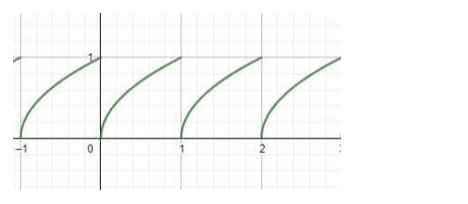# Write the domain and the range of the function,

Question:

Write the domain and the range of the function, $f(x)=\sqrt{x-[x]}$.

Solution:

Given, $\mathrm{f}(\mathrm{x})=\sqrt{x-[x]}$

Where [x] is the Greatest Integer Function of x.

$f(x)=\sqrt{\{x\}}$

Where {x} is fractional part of x.

The graph of $f(x)$ is(i) dom(f)

Domain of{x} is R.

The value of the fractional part of x is always either positive or zero.

Hence domain of f(x) is R.

(ii) range(f)

Range of $\{x\}$ is $[0,1)$.

As the root value $[0,1)$ between interval lies between $[0,1)$.

Hence range of $f(x)$ is $[0,1)$.